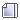###Author Topic: The Puzzle Thread!  (Read 8066 times)

0 Members and 1 Guest are viewing this topic.

####nslay

• Hero Member
••• Posts: 786
• Giraffe meat, mmm« Reply #15 on: March 18, 2011, 10:12:38 PM »
I'm pretty sure this is The Puzzle Thread not The Math Thread.  Knock it off...
I didn't know there was a difference. Do you like your math served up in words, or chopped into numbers?

Maybe that's how we can persuade people to like math ... we can rename the subject to "Puzzles" or "Solving Puzzles"!####nslay

• Hero Member
••• Posts: 786
• Giraffe meat, mmm« Reply #16 on: June 07, 2011, 12:31:31 PM »
Given two lines in 1D of lengths $\ell_1,\ \ell_2$ each centered at $x_1,\ x_2 \in \mathbb{R}$, find the overlap of the lines. For example, if $x_1 = 0,\ x_2 = \frac{1}{2}$ and $\ell_1 = \ell_2 = 1$ then the overlap should be $\frac{1}{2}$.

It has a relatively simple solution, though it does not appear to be as simple to derive as would be expected. Perhaps I'm over thinking it. I want to see if anyone can find an easier derivation.

I'll post my derivation in a day or upon seeing a derivation.

EDIT: Oh yeah, I think I found an easier way. I'll post both solutions.
« Last Edit: June 07, 2011, 01:10:40 PM by nslay »####nslay

• Hero Member
••• Posts: 786
• Giraffe meat, mmm« Reply #17 on: June 07, 2011, 05:14:30 PM »
My original solution:

My idea was to represent each line as a box car function. The integral of the box car function is the length of it's non-zero portion. Thus, the integral of the product of the two box car functions would be the length of the overlap.

So we have two lines
$\Pi_{-\ell_1/2,\ell_1/2}(x - x_1) = H(x - x_1 + \ell_1/2) - H(x-x_1-\ell_1/2) \Pi_{-\ell_2/2,\ell_2/2}(x - x_2) = H(x - x_2 + \ell_2/2) - H(x-x_2-\ell_2/2)$
where $H(\cdot)$ is the Heaviside function

Then, the overlap is given by the integral
$\text{overlap} = \int_{-\infty}^{\infty} \Pi_{-\ell_1/2,\ell_1/2}(x-x_1) \Pi_{-\ell_2/2,\ell_2/2}(x-x_2) dx$

First note that
$\frac{d}{dx} \left [ \Pi_{-\ell_1/2,\ell_1/2}(x-x_1) \right ] = \delta(x-x_1+\ell_1/2) - \delta(x-x_1-\ell_1/2) \int \Pi_{-\ell_2/2,\ell_2/2}(x-x_2) dx = (x - x_2 + \ell_2/2) I(x - x_2 + \ell_2/2 > 0) - (x - x_2 - \ell_2/2) I(x - x_2 - \ell_2/2 > 0)$
where $\delta(\cdot)$ denotes the Dirac delta function and $I(\cdot)$ denotes the indicator function.

By integrating by parts, we can simplify the integral
$\int_{-\infty}^{\infty} \Pi_{-\ell_1/2,\ell_1/2}(x-x_1) \Pi_{-\ell_2/2,\ell_2/2}(x-x_2) dx = \left . \left [ \Pi_{-\ell_1/2,\ell_1/2}(x-x_1) \int \Pi_{-\ell_2/2,\ell_2/2}(x-x_2) dx \right ] \right |_{-\infty}^{\infty} - \int_{-\infty}^{\infty} \underbrace{[ (x - x_2 + \ell_2/2) I(x - x_2 + \ell_2/2 > 0) - (x - x_2 - \ell_2/2) I(x - x_2 - \ell_2/2 > 0) ]}_{\int \Pi_{-\ell_2/2,\ell_2/2}(x-x_2) dx} \underbrace{[ \delta(x-x_1+\ell_1/2) - \delta(x-x_1-\ell_1/2) ]}_{\frac{d}{dx} \left [ \Pi_{-\ell_1/2,\ell_1/2}(x-x_1) \right ] } dx$

The first term cancels since $\Pi_{-\ell_1/2,\ell_1/2}(\pm \infty-x_1) = 0$. The second term is trivial to compute since
$\int_{-\infty}^{\infty} f(x) \delta(x-a) dx = f(a)$

This gives
$\int_{-\infty}^{\infty} \Pi_{-\ell_1/2,\ell_1/2}(x-x_1) \Pi_{-\ell_2/2,\ell_2/2}(x-x_2) dx = - (x_1 - \ell_1/2 - x_2 + \ell_2/2) I(x_1 - \ell_1/2 - x_2 + \ell_2/2 > 0) + (x_1 - \ell_1/2 - x_2 - \ell_2/2) I(x_1 - \ell_1/2 - x_2 - \ell_2/2 > 0) + (x_1 + \ell_1/2 - x_2 + \ell_2/2) I(x_1 + \ell_1/2 - x_2 + \ell_2/2 > 0) - (x_1 + \ell_1/2 - x_2 - \ell_2/2) I(x_1 + \ell_1/2 - x_2 - \ell_2/2 > 0)$

If we write $\Delta x = x_1 - x_2,\ u = \frac{\ell_1+\ell_2}{2},\ v = u - \ell_2$ then we have a more simplified form

$\text{overlap}(x_1,x_2,\ell_1,\ell_2) = - (\Delta x - v) I(\Delta x - v > 0) + (\Delta x - u) I(\Delta x - u > 0) + (\Delta x + u) I(\Delta x +u > 0) - (\Delta x + v) I(\Delta x + v > 0)$

It's a nifty looking solution. In C it would be
Code: [Select]
double x1, x2, l1, l2, dx, u, v, tmp, L;dx = x1 - x2;u = 0.5*(l1+l2);v = u - l2;tmp = dx - v;L = -tmp*(tmp > 0);tmp = dx - u;L += tmp*(tmp > 0);tmp = dx + u;L += tmp*(tmp > 0);tmp = dx + v;L -= tmp*(tmp > 0);
One could do a more direct solution by considering the end points of the lines instead of the midpoints. Then it's a lot easier to derive directly and reduces to 4 possible if conditions. That matches the 4 indicator functions the integration gives (indicators are like branchless if statements).

EDIT: Fixed some errors (Forgot to divide by 2 in some places).
« Last Edit: June 07, 2011, 11:30:27 PM by nslay »